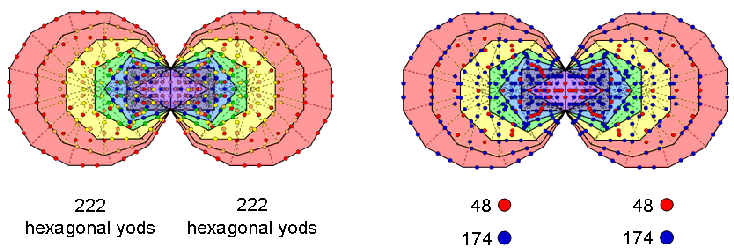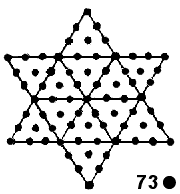<< Previous    1...   20  21    23  24  ...56    Next >>

#22 The tetrahedron & octahedron embody, respectively, the dimension 248 of E8 & the dimension 496 of E8×E8

Suppose that a geometrical object can be divided up into T triangles with C corners and S sides. When these triangles are changed into tetractyses, the number of hexagonal yods = 2S + T and the total number of yods = C + 2S + T. The numbers of corners of triangles needed to construct both the interior and faces of a polyhedron with V vertices, E edges & F faces that are all n-gons (not necessary regular) with nF sectors was tabulated in #21. The numbers of yods in its faces and interior that surround its centre are tabulated below:

 Corners Hexagonal yods Total Faces: 2 + E 2(E+nF) + nF = 2E + 3nF 2 + 3E + 3nF Interior: E + nF 2(2+4E+3nF) + 3E + 3nF = 4 + 11E + 9nF 4 + 12E + 10nF Total = 2 + 2E + nF 4 + 13E + 12nF 6 + 15E + 13nF

The number "2" refers to the two endpoints of an axis passing through the centre of the polyhedron, the number "4" refers to the four hexagonal yods on this axis and the number "6" refers to the six yods on the axis that surround its middle. For the tetrahedron, E = 6, F = 4 & n =3; for the octahedron, E = 12, F = 8 & n = 3; for the cube, E = 12, F = 6 & n = 4; for the icosahedron, E = 30, F = 20 & n = 3; for the dodecahedron, E = 30, F = 12 & n = 5. The yod populations of the five Platonic solids are tabulated below:

 Platonic solid Corners Hexagonal yods Total Tetrahedron Faces Interior Total = 2 + 6 18 2 + 24 484 + 174 4 + 222 2 + 54 4 + 192 6 + 246 Octahedron FacesInteriorTotal = 2 + 12 36 2 + 48 96 4 + 348 4 + 444 2 + 108 4 + 384 6 + 492 Cube FaceInterior Total = 2 + 12 36 2 + 48 96 4 + 348 4 + 444 2 + 108 4 + 384 6 + 492 Icosahedron Faces InteriorTotal = 2 + 30 90 2 + 120 240 4 + 870 4 + 1110 2 + 270 4 + 960 6 + 1230 Subtotal = FacesInteriorTotal = 8 + 60 180 8 + 240 480 16 + 1740 16 + 2220 8 + 540 16 + 1920 24 + 2460 Dodecahedron Faces Interior Total = 2 + 30 90 2 + 120 240 4 + 870 4 + 1110 2 + 270 4 + 960 6 + 1230 Total = Faces InteriorTotal = 10 + 90 270 10 + 360 720 20 + 2610 20 + 3330 10 + 810 20 + 2880 30 + 3690

(to clarify the display, the centre is excluded from the numbers in the table).

Tetrahedron
The Godname YAHWEH with number value 26 prescribes the tetrahedron because its 66 tetractyses have 26 corners surrounding its centre. The 222 hexagonal yods surrounding its axis comprise 48 hexagonal yods in its four faces and 174 hexagonal yods in its interior. This property demonstrates that the tetrahedron possesses properties analogous to those of the inner form of the Tree of Life, for the two sets of seven enfolded polygons contain 444 hexagonal yods, so that 222 hexagonal yods are associated with each

set(see also #24 in Wonders of correspondences). Of these, 174 hexagonal yods lie on 87 sides of the 47 tetractyses in each set and 48 hexagonal yods comprise the hexagonal yod on the root edge associated with either set and the hexagonal yods at the centres of its 47 tetractyses. The table indicates that 246 yods surround the axis of the tetrahedron, where 246 is the number value of Gabriel, the Archangel of Yesod. 174 internal hexagonal yods surround the axis, 87 hexagonal yods in each half. 87 is the number value of Levanah, the Mundane Chakra of Yesod. As seven yods lie on the axis, 252 yods surround the centre of the tetrahedron. 248 yods are either in the faces (56) or inside the tetrahedron (192) surrounding the axis. They symbolize the 248 roots of the rank-8 Lie group E8 at the heart of superstring theory. The four vertices and the centres of the four faces denote its eight simple roots. The 48 hexagonal yods in the faces and the 192 internal yods surrounding the axis denote the 240 roots of E8.

Octahedron
It has 50 corners, 48 surrounding its axis. 12 of the latter are in its faces and 36 are internal. This 2:12:36 pattern corresponds in the inner Tree of Life to the 2 endpoints of the root edge, to the 12 corners of the dodecagon and the 36 corners of the first six separate polygons:Alternatively, the pattern corresponds to the two hexagonal yods in the root edge, the 12 hexagonal yods at centres of the tetractys sectors of the dodecagon and the 36 hexagonal yods at the centres of the tetractyses making up the first six polygons. The 96 hexagonal yods in the faces correspond to the 96 hexagonal yods on the sides of the seven separate polygons. 492 yods surround the axis, 246 in each half. This means that, other than two diametrically opposite vertices, (248+248=496) yods surround the centre of the polyhedron. The octahedron embodies the root composition of E8×E8 that is at the heart of one of the two heterotic superstring theories. Surrounding its axis are 444 hexagonal yods This is the polyhedral counterpart to the 444 hexagonal yods in the (7+7) enfolded polygons of the inner Tree of Life (see the picture shown above in the section on the tetrahedron).

Cube
As the cube is the dual of the octahedron, it has the same number of edges. Its value of nF is the same as that for the octahedron, so the formulae given above generate the same set of numbers. However, its 96 hexagonal yods correspond to the 96 hexagonal yods lining the internal sides of the tetractyses making up the seven separate polygons of the inner Tree of Life.

Icosahedron
120 corners surround its axis, where 120 = 22 + 42 + 62 + 82 = 112 − 1

 3 5 7 = 9 11 13 15 17 19 21 ,

showing how it is prescribed by EHYEH, the Godname of Kether with number value 21. Its eight faces have 240 hexagonal yods, where 240 = 1×2×3×4(1+2+3+4). This is the number of hexagonal yods in the seven separate polygons of the inner Tree of Life. Its faces have 272 yods, where 272 is the number value of Cherubim, the Order of Angels assigned to Yesod. 870 (= 87×10) internal yods surround its axis, where 87 is the number value of Levanah, the Mundane Chakra of Yesod. 1230 (= 123×10) yods surround its axis, where 123 is the tenth Lucas number (Lucas numbers are defined in Fig. 4 of Article 50 (Part 1)).

Dodecahedron
As the dodecahedron is the dual of the icosahedron, it has the same number of edges and its value of nF is the same as that for the icosahedron. That is why the formulae given above generates the same set of numbers. However, the 240 hexagonal yods in its faces correspond to the 240 hexagonal yods in the the second set of seven separate polygons of the inner Tree of Life. Duality of the icosahedron and dodecahedron corresponds to the mirror symmetry between the two sets of polygons. (See also #14 and #15 at Sacred geometry/Platonic solids).

The first four Platonic solids
The 38 faces have 548 yods. The average number of yods needed to construct them = 548/4 = 137. This is the number whose reciprocal determines the approximate value of the fine-structure constant (see also #16 in Sacred geometry/Platonic solids). It was shown in #20 to be the number of corners & triangles needed on average to create half a Platonic solid, the average being made over all five solids.

The five Platonic solids
The 180 triangular sectors in their 50 faces have 100 corners, where 100 = 13 + 23 + 33 + 43. 50 corners and 360 (= 36×10) hexagonal yods are in the 25 faces in the set of five of the ten halves of the solids. This shows how the Godnames of Binah and Geburah on the Pillar of Judgement with, respectively, number values 50 & 36, prescribe the five regular polyhedra. The five solids also have 360 corners surrounding their axes, of which there are (360 – 50 = 310 = 31×10) corners other than their vertices. On average, 31 such corners are in each half of a Platonic solid. This shows how EL, the Godname of Chesed with number value 31, prescribes the five Platonic solids. 155 corners other than vertices surround their axes in each half. This shows how ADONAI MELEKH, the Godname of Malkuth with number value 155, prescribes the geometry of the five Platonic solids. ADONAI with number value 65 separately prescribes them because there are a total of 370 corners of triangles, i.e., 375 corners when their centres are included, so that they have (375 – 50 = 325) corners other than vertices, an average of 65 such corners.

The five Platonic solids have 3350 hexagonal yods. On average, they have 670 (= 67×10) hexagonal yods. 67 is the number value of Binah. As pointed out in #10, it is the number of yods below Binah of the 1-tree. This property of the five Platonic solids is an amazing illustration of how the system of Sephirothic names, Godnames, etc define properties of holistic systems. This is confirmed by the following: the total number of yods surrounding the centres of the five Platonic solids is 3720. The average number of such yods = 3720/5 = 744. As this includes the average number of 10 vertices, as well as the four hexagonal yods in the axis, on average there are (744 − 10 − 4 = 730 = 73×10) yods other than vertices surrounding the axes of the five Platonic solids, where 73 is the number value of Chokmah. There are 73 yods up to Chokmah of the 1-tree (see #10). The appearance of the numbers of both Chokmah & Binah in determining the average yod population of the five Platonic solids refutes the suggestion that it could be due to chance. Moreover, the construction of the Star of David/Sign of Vishnu from tetractyses requires 73 yods (67 other than the six yods at the tips of the star):This reveals one of the amazing properties of this ancient, religious image because, if the Decad (10) is assigned to each yod in the 12 tetractyses needed to construct it, their sum (730) is the average number of yods surrounding their axes that are needed to construct the five Platonic solids from tetractyses, given just their vertices, whilst the sum (670) of all 10s other than those at the tips is 670, which is the average number of hexagonal yods in the five Platonic solids, including those in their axes, which number 4 on average. Excluding the latter, the average number of hexagonal yods surrounding the axis of a Platonic solid = 670 − 4 = 666. Perhaps the less said about this number, the better! However, it must be pointed out that 666 is the 36th triangular number:

1 + 2 + 3 + .... + 36 = 666.

This shows how ELOHA, the Godname of Geburah with number 36, prescribes the average population of hexagonal yods making up the five Platonic solids.

 << Previous    1...   20  21    23  24  ...56    Next >>

Home